Question

# CSC Hw Problems. Any help is appreciated I dont know where to start let alone what...

CSC Hw Problems. Any help is appreciated I dont know where to start let alone what the answers are.

Your assignment is to write your own version of some of the functions in the built-in <string.h> C library. As you write these functions, keep in mind that a string in C is represented as a char array, with the '\0' character at the end of the
string. Therefore, when a string is passed as a parameter, the length of the string does not necessarily need to be passed as a separate parameter. The string itself may or may not completely fill the array.
Note that the main function that I have provided does use <string.h> as it constructs test strings to pass to your functions. However, your solutions for the 5 functions below may not use any of the built-in C string functions from the <string.h> library.
1. Write a function called strcmp373. This function is passed two parameters, both of which are C strings. You should use array syntax when writing this function; that is, you may use [ ], but not * or &. The function should return an int as follows:
a. A negative number if the first string is alphabetically before the second string. In C, the return value of strcmp is a number which reflects the difference in the ASCII codes for the first 2 letters which are not the same. For example, a call to strcmp("programming", "project") returns -3, since 'g' - 'j' in ASCII is -3. b. Zero if the strings are the same c. A positive number if the second string is alphabetically before the first string. Again, the number is the difference in ASCII codes between the first 2 letters which are not the same.
Here are some examples of how your code should work:
strcmp373("bin", "bag"); // returns 8 strcmp373("computer", "game"); // returns -4 strcmp373("computer", "computer"); // returns 0 strcmp373("are", "area"); // returns -97, because '\0'- ‘a’ = 0-97 = 97.
2. Write a function called strcat373. The function is passed two parameters, both of which are C strings. You should use pointer syntax when writing this function (in other words, you can use * and &, but not [ ]). The function should modify the first parameter (a char array, often called the destination array) so that it contains the concatenation of the two strings, and returns a pointer to the destination array. For example:
int main() { char str1 = "comp"; char str2[ ] = "uter"; char *str3; strcat373(str1, str2); // prints computer twice printf("str1 contains %s\nstr3 contains %s\n, str1, str3);

Note that strcat373 is guaranteed to work properly only if str1's length is big enough to contain the concatenation of the two strings. In this case, "computer" takes up 9 bytes, and since str1 is 9 bytes, the function should run properly since the array is just long enough to contain “computer” (plus ‘\0’). On the other hand : char str1[] = "comp"; // only 5 bytes char str2[] = "uter"; strcat373(str1, str2); // takes up 9 bytes and // therefore overflows str1
Upon execution, a runtime error may occur, or (even worse) no runtime error will occur, but some other variable(s) in your program may be overwritten.
3. Write a function called strchr373. It is passed 2 parameters: a string and a char Here is the prototype for the function:
char *strchr373(char str[], char ch);
The function should return a pointer to the first instance of ch in str. For example:
int main { char s[ ] = "abcbc"; printf("%s", strchr373(s, 'b')); // prints bcbc printf("%s", strchr373(s, 'c'); // prints cbc printf("%d", strchr373(s, 'd'); // prints 0
4. Write a function called strncpy373. It is passed 3 parameters: 2 strings and the length of the destination array (the first parameter). The intent of including a third parameter is to prevent overflow of the destination array in case the source array (the second parameter) contains a string that is too long to be stored in the destination. strncpy373 returns a pointer to the destination array.
For example:
char s1[] = "comp"; char s2[] = "systems"; strncpy373(s1, s2, 4); printf("%s\n", s1);
The output of the above code should be syst.
5. Write a function called strncat373. Similarly to the previous functions, if properly used strncat373 prevents overflow of the destination array in case the source array (the second parameter) contains a string that is too long to be stored in the destination. As the function’s name indicates, this time
concatenation is performed on the source string rather than copying (and overwriting) that string.

Main Function:

#include <stdio.h>

#include <string.h>

#include "hw2.c"

int main() {

char word1 = "bin",

word2[] = "bag",

word3 = "computer",

word4[] = "game",

word5[] = "area",

word6[] = "area",

word7[] = "are",

word8 = "bo",

word9[] = "ringer";

int cmp1 = strcmp373(word1, word2),

cmp2 = strcmp373(word3, word4),

cmp3 = strcmp373(word5, word6),

cmp4 = strcmp373(word6, word7);

printf("Test strcmp373\n");

printf("%s %s = %d\n", word1, word2, cmp1);

printf("%s %s = %d\n", word3, word4, cmp2);

printf("%s %s = %d\n", word5, word6, cmp3);

printf("%s %s = %d\n", word6, word7, cmp4);

printf("\nTest strcat373\n");

printf("%s + %s = ", word1, word2);

printf("%s\n", strcat373(word1, word2));

printf("%s + \" \" + %s = ", word3, word4);

strcat373(word3, " ");

strcat373(word3, word4);

printf("%s\n", word3);

printf("%s + %s = ", word8, word7+1);

printf("%s\n", strcat373(word8, word7+1));

printf("\nTest strchr373\n");

char s[ ] = "abcbc";

printf("strchr373(%s,%c) = ", s, 'b');

printf("%s", strchr373(s, 'b')); // prints bcbc

printf("\n");

printf("strchr373(%s,%c) = ", s, 'c');

printf("%s\n", strchr373(s, 'c')); // prints cbc

printf("\n");

printf("strchr373(%s,%c) = ", s, 'd');

printf("%d", strchr373(s, 'd')); // prints 0

printf("\n");

printf("\nTest strncpy373\n");

char s1[] = "comp";

char s2[] = "systems";

strncpy(s1, s2, 4);

printf("%s\n", s1);

printf("\nTest strncat373\n");

printf("%s + %s %d = ", word8, word9, 4 );

strncat373(word8, word9, 4);

printf("%s\n", word8);

}

```#include <stdio.h>

int strcmp373(char *a, char *b) {
int i=0;
while(1) {
if(a[i] == '\0' && b[i] == '\0') {
// both strings are same
return 0;
}
// some unmatch character found
if(a[i] != b[i]) {
return a[i] - b[i];
}
// move to next character
i++;
}
}

char * strcat373(char *a, char *b) {
int i = 0;
// find end of string 1
while(*(a+i) != '\0') {
i++;
}
int j = 0;
while(*(b+j) != '\0') {
*(a+i) = *(b+j);
j++;
i++;
}
a[i] = '\0';
return a;
}

char *strchr373(char str[], char ch) {
int i = 0;
// find end of string 1
while(str[i] != '\0') {
if(str[i] == ch) {
return &str[i];
}
i++;
}
return NULL;
}

void strncpy373(char *a, char *b, int n) {
int j = 0;
while((*(b+j) != '\0') && (j < n)) {
*(a+j) = *(b+j);
j++;
}
b[j] = '\0';
}

void strncat373(char *a, char *b, int n) {
int i = 0;
// find end of string 1
while(*(a+i) != '\0') {
i++;
}
int j = 0;
while((*(b+j) != '\0') && (j < n)) {
*(a+i) = *(b+j);
j++;
i++;
}
a[i] = '\0';
}

int main() {

char word1 = "bin",

word2[] = "bag",

word3 = "computer",

word4[] = "game",

word5[] = "area",

word6[] = "area",

word7[] = "are",

word8 = "bo",

word9[] = "ringer";

int cmp1 = strcmp373(word1, word2),

cmp2 = strcmp373(word3, word4),

cmp3 = strcmp373(word5, word6),

cmp4 = strcmp373(word6, word7);

printf("Test strcmp373\n");

printf("%s %s = %d\n", word1, word2, cmp1);

printf("%s %s = %d\n", word3, word4, cmp2);

printf("%s %s = %d\n", word5, word6, cmp3);

printf("%s %s = %d\n", word6, word7, cmp4);

printf("\nTest strcat373\n");

printf("%s + %s = ", word1, word2);

printf("%s\n", strcat373(word1, word2));

printf("%s + \" \" + %s = ", word3, word4);

strcat373(word3, " ");

strcat373(word3, word4);

printf("%s\n", word3);

printf("%s + %s = ", word8, word7+1);

printf("%s\n", strcat373(word8, word7+1));

printf("\nTest strchr373\n");

char s[ ] = "abcbc";

printf("strchr373(%s,%c) = ", s, 'b');

printf("%s", strchr373(s, 'b')); // prints bcbc

printf("\n");

printf("strchr373(%s,%c) = ", s, 'c');

printf("%s\n", strchr373(s, 'c')); // prints cbc

printf("\n");

printf("strchr373(%s,%c) = ", s, 'd');

printf("%d", strchr373(s, 'd')); // prints 0

printf("\n");

printf("\nTest strncpy373\n");

char s1[] = "comp";

char s2[] = "systems";

strncpy373(s1, s2, 4);

printf("%s\n", s1);

printf("\nTest strncat373\n");

printf("%s + %s %d = ", word8, word9, 4 );

strncat373(word8, word9, 4);

printf("%s\n", word8);

}```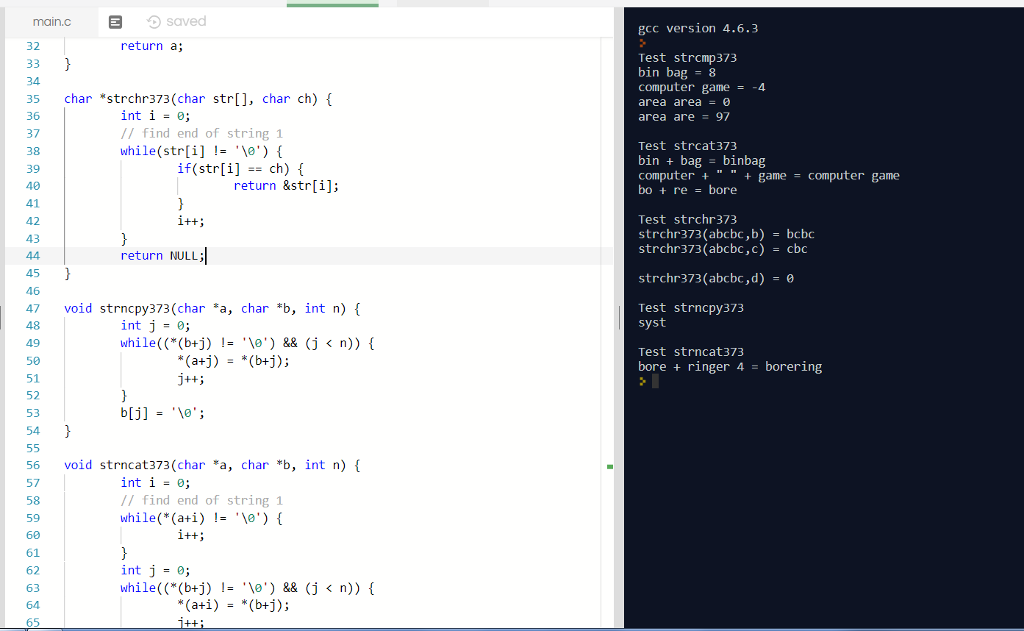#### Earn Coins

Coins can be redeemed for fabulous gifts.

Similar Homework Help Questions
• ### write C code that uses pointers, arrays, and C strings. 3. Write a function called pow_xy....

write C code that uses pointers, arrays, and C strings. 3. Write a function called pow_xy. The function should be passed 2 parameters, as illustrated in the prototype below. int pow_xy(int *xptr, int y); Assuming that xptr contains the address of variable x, pow_xy should compute x to the y power, and store the result as the new value of x. The function should also return the result. Do not use the built-in C function pow. For the remaining problems,...

• ### SCREENSHOTS ONLY PLEASE!!! DON'T POST ACTUAL CODE PLEASE LEAVE A SCREENSHOT ONLY! ACTUAL TEXT IS NOT NEEDED!!! myst...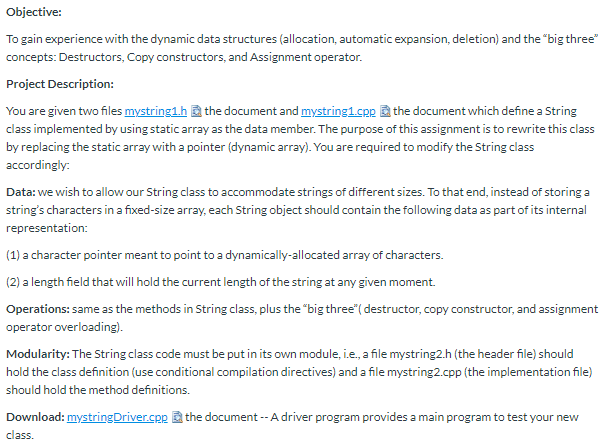SCREENSHOTS ONLY PLEASE!!! DON'T POST ACTUAL CODE PLEASE LEAVE A SCREENSHOT ONLY! ACTUAL TEXT IS NOT NEEDED!!! mystring.h: //File: mystring1.h // ================ // Interface file for user-defined String class. #ifndef _MYSTRING_H #define _MYSTRING_H #include<iostream> #include <cstring> // for strlen(), etc. using namespace std; #define MAX_STR_LENGTH 200 class String { public: String(); String(const char s[]); // a conversion constructor void append(const String &str); // Relational operators bool operator ==(const String &str) const; bool operator !=(const String &str) const; bool operator >(const...

• ### Please answer problem #5 thank you str.c #include "str.h" #include <stdio.h> int str_len(...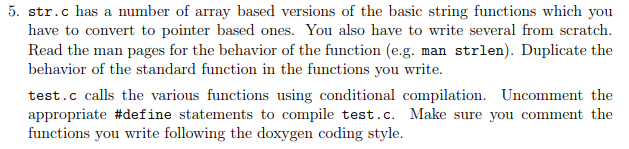Please answer problem #5 thank you str.c #include "str.h" #include <stdio.h> int str_len(char *s) {    /* this is here so code compiles */ return 0; } /* array version */ /* concantenate t to the end of s; s must be big enough */ void str_cat(char s[], char t[]) {    int i, j;    i = j = 0;    while (s[i] != '\0')    /* find end of s */        i++;    while ((s[i++] = t[j++]) != '\0') /* copy t */        ;...

• ### Please use Visual Studio! Let me know if you need anything else <3 #include <stdio.h> #include...

Please use Visual Studio! Let me know if you need anything else <3 #include <stdio.h> #include <string.h> #pragma warning(disable : 4996) // compiler directive for Visual Studio only // Read before you start: // You are given a partially complete program. Complete the functions in order for this program to work successfully. // All instructions are given above the required functions, please read them and follow them carefully. // You shoud not modify the function return types or parameters. //...

• ### Hi, it's C++ question. Only can use <iostream> and <fstream>libraries Please help me, thanks Question Description:...

Hi, it's C++ question. Only can use <iostream> and <fstream>libraries Please help me, thanks Question Description: For this project you will write a program to: a) read-in the 10 first names from a file (the file is a priori given to have exactly 10 entries, of a maximum length of 8 letters each) into a 2-dimensional character array, b) output the names to the terminal with each one preceded by a number indicating its original order in the list, c)...

• ### Hey everyone, I need help making a function with this directions with C++ Language. Can you...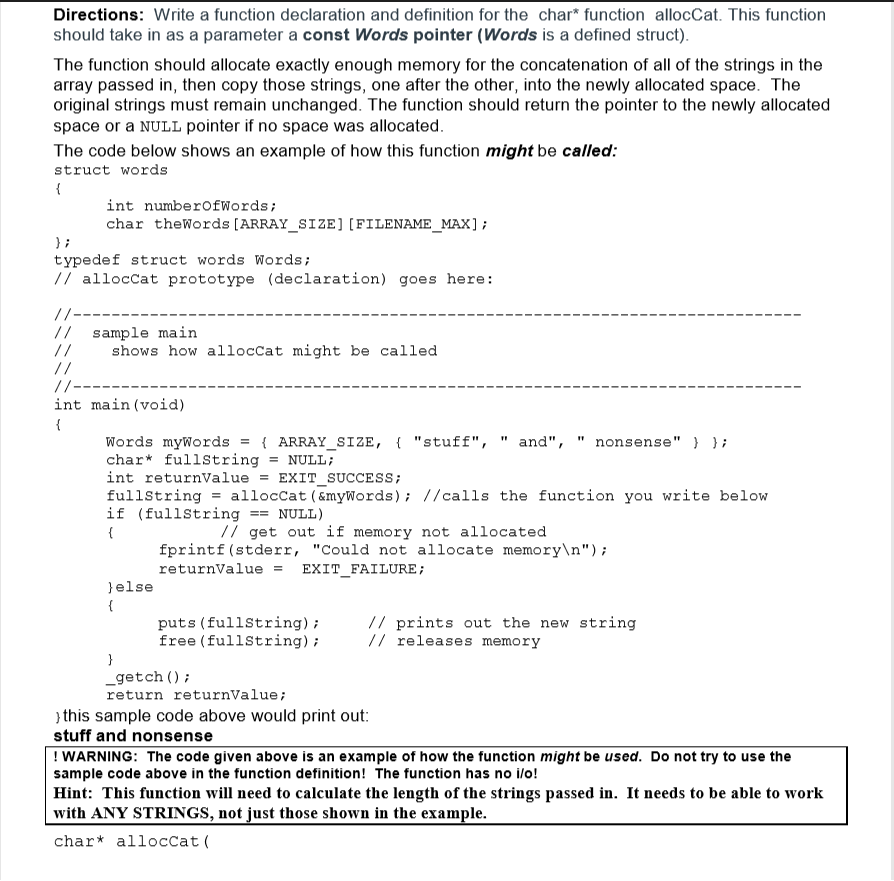Hey everyone, I need help making a function with this directions with C++ Language. Can you guys use code like printf and fscanf without iostream or fstream because i havent study that yet. Thanks. Directions: Write a function declaration and definition for the char* function allocCat. This function should take in as a parameter a const Words pointer (Words is a defined struct) The function should allocate exactly enough memory for the concatenation of all of the strings in the...

• ### Need help coding these two C programs 1:27 LTE < s3?bucket-uploads&prefix-attach%2Fjl CS 100 Exam Two -Coding...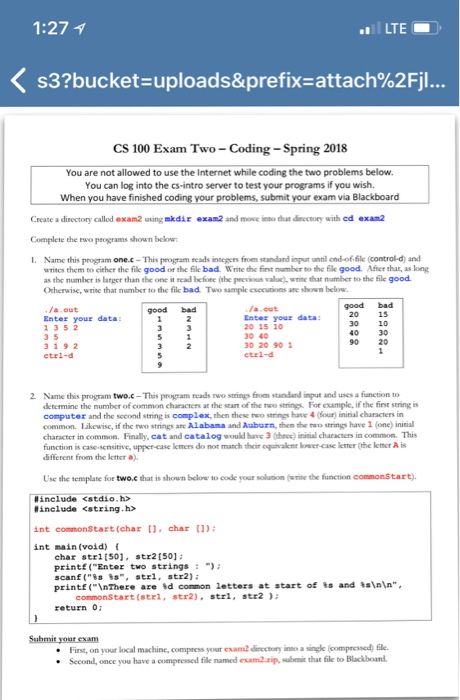Need help coding these two C programs 1:27 LTE < s3?bucket-uploads&prefix-attach%2Fjl CS 100 Exam Two -Coding -Spring 2018 You are not allowed to use the Internet while coding the two problems below. You can log into the cs-intro server to test your programs if you wish When you have finished submit your exam via Blackboard Create a directory called exam2 using mkdir exan2 and move into that dinectory with ed exan2 Complete the I. Name this program one.c-This peogram reads...

• ### T/F C Language Questions. Answer the following true/false questions. You must correctly state WHY your answer...

T/F C Language Questions. Answer the following true/false questions. You must correctly state WHY your answer is true or false in order to receive credit. #include <stdio.h> #include <string.h> int run_through(int num, char **a) { int i; int check=0; for(i=0;i<num;i++) { printf("%s\n", *(a+i)); if(strcmp(*(a+i), "filename")==0) { check=1; } } return check; } char** find_filename(int n, char **b) { int i; int check=0; for(i=0;i<n;i++) { if(strcmp(*b, "filename")==0) { b++; break; } b++; } return b; } int main(int argc, char **argv)...

• ### I need this in C not C++ just C SET-151 C Programming #1 Homework: 7 String...

I need this in C not C++ just C SET-151 C Programming #1 Homework: 7 String Basics – Death by a thousand strings.                                                                                                                                                                         Reading(s): Chapter(s) in C Primer Plus 1-7, 9, 10 please use this format // ------------------------------------------------------------------------------------------ // Name: Your name here // Class: C Programming // Abstract: Homework 1. // ------------------------------------------------------------------------------------------ // ------------------------------------------------------------------------------------------ // Includes // ------------------------------------------------------------------------------------------ #include<stdio.h> #include<stdlib.h> // ------------------------------------------------------------------------------------------ // Constants // ------------------------------------------------------------------------------------------ // ------------------------------------------------------------------------------------------ // Prototypes // ------------------------------------------------------------------------------------------ // ------------------------------------------------------------------------------------------ // Name: main //...

• ### // READ BEFORE YOU START: // You are given a partially completed program that creates a...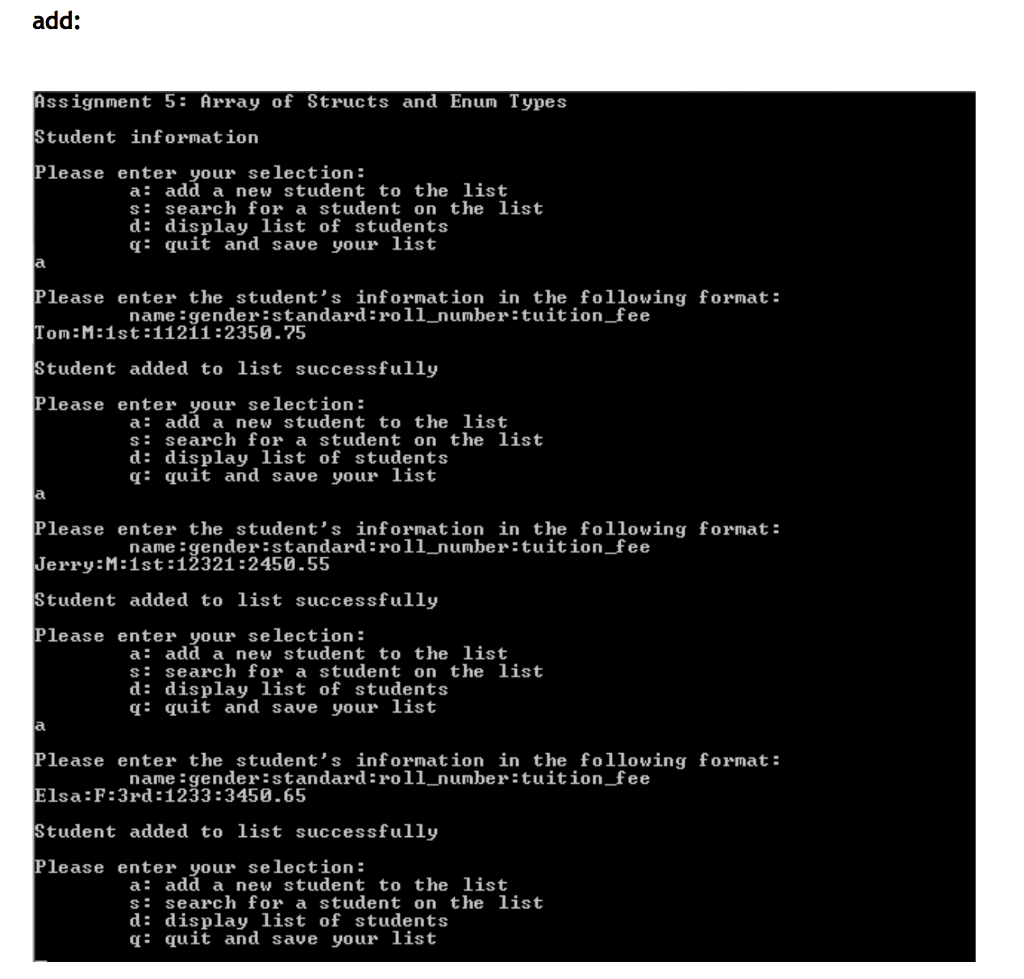// READ BEFORE YOU START: // You are given a partially completed program that creates a list of students for a school. // Each student has the corresponding information: name, gender, class, standard, and roll_number. // To begin, you should trace through the given code and understand how it works. // Please read the instructions above each required function and follow the directions carefully. // If you modify any of the given code, the return types, or the parameters, you...